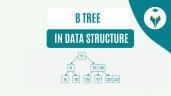clickHere
Updated on Dec 26, 2022 13:59 IST

Circular Linked Lists traverse in the form of a circle. You can begin at any node and traverse the list in either the forward or backward direction to reach the same node again. In this article, let us understand the depths of Circular Linked Lists in the following order:

## What is Circular Linked List

As the name suggests, Circular Linked Lists traverse in the form of a circle. So, you can begin at any node and traverse the list in either the forward or backward direction until you reach the same node again. The last node of the Circular Linked Lists contains the pointer to the first node of the list. Hence, there is no start or endpoint of this type of list.

Note:

• A Circular Linked List can be Singly if the next pointer of the last item points to 1st item in the list
• A Circular Linked List can be Doubly if the previous pointer of 1st  item points to the last item in the list

A few of the most popular applications of Circular Linked Lists are:

• Multiplayer games
• Systems where multiple applications are running

### Representation of Circular Linked Lists

Circular Singly Linked List is a chain of nodes, and each node has the following parts:

• Data Item
• Address to the next node

Node is represented in the C programming language as follows:

struct node
{
int d;
struct node *n;
};

Node is represented in Python programming language as follows:

class Node:

def __init__(self, d):
self.d = d
self.n = None

Circular Doubly Linked List is a chain of nodes, and each node has the following parts:

• Data Item
• Address to the next node

Node is represented in the C programming language as follows:

struct node
{
int d;
struct node *n;
struct node *p;
};

Node is represented in Python programming language as follows:

class Node:

def __init__(self, d):
self.d = d
self.n = None
self.p = None

### Traversal

Traversal refers to accessing elements in the list and displaying them as output.

To traverse through the list, you have to keep moving the temp node to the next node until the temp is pointed towards the 1st node. As soon as temp = 1st node, it implies that you have completed your traversal.

### Insertion

Insertion for Singly Circular Linked Lists

There are 3 ways to insert elements in Circular Singly Linked Lists.

Note: For all the types of insertion, you have to allocate memory and store data for the new node

• Insert at the beginning
•  Initially, store the address of the current 1st node to the new node
• Then, point the last node to the new node. Thus, making the new node as head.
• Insert after a node
• Go to the node after which the new node has to be inserted. Let us consider this as X
• Point the next of new node to the node next to the node next to X
• Store the address of the new node at the next of X
• Insert at the end
• Store the address of the head node to the next of new node, thus making the new node the LAST node
• Point the current last node to new node
• Finally, make the new node as the last node

### Deletion

Deletion for Singly Circular Linked Lists

There are 3 ways to delete elements in Singly Circular Linked Lists.

To delete

For the only node present, you have to free the memory occupied by the node and put NULL in the last node.

After a particular key element, you have to go to the node which has to be deleted and store the address previous to this one in the node next to the one which has to be deleted.

For the last node, you have to store the address of the node previous to the last node in the 1st node. Then, free the memory of the node which has to be deleted.

## Circular Linked Lists in C

Refer to the following code to understand how to create Circular Linked Lists and perform various operations in C.

` `
`//Circular Singly Linked List in C#include <stdio.h>#include <stdlib.h>struct node {  int d;  struct node* n;}; struct node* EmptyList(struct node* end, int d) {  if (end != NULL) return end;   struct node* newnode = (struct node*)malloc(sizeof(struct node));  newnode->d = d;  end = newnode;  end->n = end;  return end;}//Traversevoid DisplayList(struct node* end) {  struct node* p;  if (end == NULL) {  printf("Sorry! Empty List");  return;  }  p = end->n;  do {  printf("%d ", p->d);  p = p->n;  } while (p != end->n);} // Insert at Beginningstruct node* InserBeg(struct node* end, int d) {  if (end == NULL) return EmptyList(end, d);  struct node* newnode = (struct node*)malloc(sizeof(struct node));  newnode->d = d;  newnode->n = end->n;  end->n = newnode;  return end;} // Insert at Endstruct node* InserEnd(struct node* end, int d) {  if (end == NULL) return EmptyList(end, d);  struct node* newnode = (struct node*)malloc(sizeof(struct node));  newnode->d = d;  newnode->n = end->n;  end->n = newnode;  end = newnode;  return end;} // Insert after a nodestruct node* InserAf(struct node* end, int d, int item) {  if (end == NULL) return NULL;  struct node *newnode, *p;  p = end->n;  do {  if (p->d == item) {    newnode = (struct node*)malloc(sizeof(struct node));    newnode->d = d;    newnode->n = p->n;    p->n = newnode;    if (p == end) end = newnode;    return end;  }   p = p->n;  } while (p != end->n);   printf("\nSorry! Node not present in the list");  return end;} // Delete a nodevoid DeleteNode(struct node** end, int key) {  if (*end == NULL) return;  if ((*end)->d == key && (*end)->n == *end) {  free(*end);  *end = NULL;  return;  }   struct node *temp = *end, *d;  if ((*end)->d == key) {  while (temp->n != *end) temp = temp->n;  temp->n = (*end)->n;  free(*end);  *end = temp->n;  }  while (temp->n != *end && temp->n->d != key) {  temp = temp->n;  }  if (temp->n->d == key) {  d = temp->n;  temp->n = d->n;  free(d);  }} int main() {  struct node* end = NULL;   end = EmptyList(end, 42);  end = InserEnd(end, 31);  end = InserBeg(end, 62);  end = InserBeg(end, 12);  end = InserEnd(end, 85);  end = InserAf(end, 10, 42);  printf("Linked List after inserting elements : \n");  DisplayList(end);  printf("\n");  DeleteNode(&end, 31);  printf("\n");  printf("Linked List after deleting elements : \n");  DisplayList(end);   return 0;}Copy code`

## Circular Linked List in Python

Refer to the following code to understand how to create Circular Linked Lists and perform various operations in Python.

` `
```//Circular Singly Linked List in Pythonclass node:  def __init__(self, d):    self.d = d    self.n = None    class CircularLL:  def __init__(self):    self.head = None      #Traverse & Display  def DisplayList(self):    x = self.head    if(x != None):      print()      print("Final Circular Linked List : ", end="  ")      print()      while (True):        print(x.d, end=" ")        x = x.n        if(x == self.head):          break      print()    else:      print("The list is empty.")          #Insert at beginning  def InserBeg(self, ele):    newnode = node(ele)    if(self.head == None):      self.head = newnode      newnode.n = self.head      return    else:      x = self.head      while(x.n != self.head):        x = x.n      x.n = newnode      newnode.n = self.head      self.head = newnode   #Insert at end  def InserEnd(self, ele):    newnode = node(ele)    if(self.head == None):      self.head = newnode      newnode.n = self.head      return    else:      x = self.head      while(x.n != self.head):        x = x.n      x.n = newnode      newnode.n = self.head         #Inserts a new element at the given pos  def InserAf(self, ele, pos):         newnode = node(ele)    x = self.head    count = 0    if(x != None):      count += 1      x = x.n    while(x != self.head):      count += 1      x = x.n        if(pos < 1 or pos > (count+1)):      print("\nInavalid pos.")    elif (pos == 1):      if(self.head == None):        self.head = newnode        self.head.n = self.head      else:        while(x.n != self.head):          x = x.n        newnode.n = self.head        self.head = newnode        x.n = self.head    else:      x = self.head      for i in range(1, pos-1):        x = x.n       newnode.n = x.n      x.n = newnode   #Delete at beginning  def DelBeg(self):    if(self.head != None):      if(self.head.n == self.head):        self.head = None      else:        x = self.head        node1 = self.head        while(x.n != self.head):          x = x.n        self.head = self.head.n        x.n = self.head         node1 = None    #Delete after an element  def DelAf(self, pos):         key = self.head    x = self.head    count = 0     if(x != None):      count += 1      x = x.n    while(x != self.head):      count += 1      x = x.n     if(pos < 1 or pos > count):      print("\nInavalid pos.")    elif (pos == 1):      if(self.head.n == self.head):        self.head = None      else:        while(x.n != self.head):          x = x.n        self.head = self.head.n        x.n = self.head        key = None     else:      x = self.head      for i in range(1, pos-1):        x = x.n       key = x.n      x.n = x.n.n      key = None        #Delete last node   def DelEnd(self):    if(self.head != None):      if(self.head.n == self.head):        self.head = None      else:        x = self.head        while(x.n.n != self.head):          x = x.n        endnode = x.n        x.n = self.head        endnode = None         # test the code                  C_ll = CircularLL() #Insert at BeginningC_ll.InserBeg(72)C_ll.InserBeg(21)C_ll.InserBeg(99)C_ll.DisplayList() #Insert at EndC_ll.InserEnd(11)C_ll.InserEnd(22)C_ll.InserEnd(32)C_ll.InserEnd(45)C_ll.DisplayList() #Insert after an elementC_ll.InserAf(59, 2)C_ll.DisplayList() #Delete at BeginningC_ll.DelBeg()C_ll.DisplayList()   #Delete after an elementC_ll.DelAf(3)C_ll.DisplayList() #Delete at endC_ll.DelEnd()C_ll.DisplayList()Copy code```

### Output:

With this, we end this article on Circular Linked Lists and how to implement them. We hope you found it informative.

Top Trending Tech Articles:
Career Opportunities after BTech | Online Python Compiler | What is Coding | Queue Data Structure | Top Programming Language | Trending DevOps Tools | Highest Paid IT Jobs | Most In Demand IT Skills | Networking Interview Questions | Features of Java | Basic Linux Commands | Amazon Interview Questions

Recently completed any professional course/certification from the market? Tell us what liked or disliked in the course for more curated content.

clickHere

This is a collection of insightful articles from domain experts in the fields of Cloud Computing, DevOps, AWS, Data Science, Machine Learning, AI, and Natural Language Processing. The range of topics caters to upski... Read Full Bio

## Trending Technology CoursesPython Foundation with Data Structures & AlgorithmsPython Foundation with Data Structures & Algorithm...
Coding ninjas4.7Algorithmic Thinking (Part 1)
Coursera4.6Data Structures and Performance
Coursera4.5Starts todaySimulation, Algorithm Analysis, and Pointers
Coursera4.4Starts todaySolving Algorithms for Discrete Optimization
Coursera4.8Starts today

## Top Picks & New Arrivals